# Diffraction

Diffraction allows to determine orientation from a set of diffraction patterns.

## Introduction¶

Determining orientation from diffraction patterns requires to collect and identify a set of diffraction vectors $\vec{q}$. When using this approach, we assume that the diffraction vector is close to a Bragg condition, which is fulfill typically in a two-beam condition. This often needs to tilt the specimen. Alternatively we present in Kikuchi a method based on Kikuchi lines.

Consider an electronic wave scattered by a crystal plane $(h,k,l)$ corresponding to a diffraction vector $\vec{q}$. Its amplitude and phase is given by:

\Psi(\vec{q})=\sum_{j=1}^N f_j e^{- 2i\pi \vec{q}\cdot\vec{r}_j}

where the sum is made on all the atoms $j$ placed at $\vec{r}_j$ in the unit cell.

The intensity recorded is thus:

I(\vec{q})=\Psi(\vec{q})\Psi^\ast(\vec{q})

For a given crystal, the wave intensity will depend both on the $\vec{q}\cdot\vec{r}$ and on the atomic form factor $f_j$.

Systematic extinctions

Extinctions for specific $(h,k,l)$ diffracted planes are expected if: $$\sum_{j=1}^N e^{- 2i\pi (h \cdot x_j + k \cdot y_j + l \cdot z_j)}=0$$ For fcc cubic crystals, with atoms placed at $(x_j,y_j,z_j)$ = $(0, 0, 0)$, $(0.5, 0.5, 0)$ ,$(0, 0.5, 0.5)$, $(0.5, 0, 0.5)$, this condition is fulfilled when $(h,k,l)$ are of different parity.

The atomic form factor $f_j$ corresponds to the amplitude of the wave diffracting on a single atom. In the range of scattering vectors between $0\leq q \leq 25 \mathring{A}^{-1}$, the atomic form factor can be approximated by a Gaussian decomposition:

f(q)=\sum _{i=1}^{4}a_{i}\exp \left(-b_{i}\left({\frac {q}{4\pi }}\right)^{2}\right)+c

where the values of $a_i$, $b_i$, and $c_i$ can be found for every atoms for instance here

The position and the name of atoms are defined in the space_group.txt file following the scheme:

Name
Atom name1 x1 y1 z1
Atom name1 x2 y2 z2
...
Atom name2 x3 y3 z3


The atomic factor is defined in the scattering.txt file according to the scheme:

Atom name1 a1 b1 a2 b2 a3 b3 a4 b4 c
...


## Diffraction pattern in TEM¶

When a plane electronic wave is scattered by a crystal under a given orientation, a spot diffraction pattern is formed in the back focal plane of the objective lens. The spots correspond to different diffraction vectors $\vec{q}$, the intensity of the pattern depending both on the crystal lattice and its orientation (see stereoproj). In Diffraction, we only use the structure factor as a guide to identify diffraction spots.

Thus, an electron wave traveling in the direction $-z$ will be diffracted by an angle $2\theta_B$ given by the Bragg law:

2d \sin{\theta_B} = n \lambda

$\lambda$ being the wavelength, $d$ the inter-reticular spacing and $n$ the diffraction order (corresponding to parallel diffraction vectors $n(h,k,l)$).

Diffracted spots will be located at a given distance $r$ from the transmitted beam and at a given angle $\eta$ from a fixed direction, chosen here as the $y$ axis.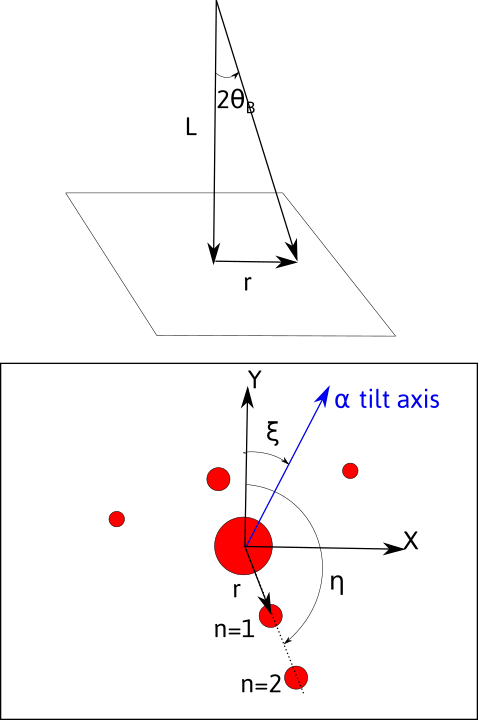The observed/recorded pattern will depend on:

• the magnification due to intermediate and projection lenses. The distance $r$ measured will thus depend on the value of $L$ called the camera length, given by $$L=\frac{rd}{n\lambda}$$ As a consequence, the pattern will depend on the acceleration voltage, through the wavelength.

• the rotation, by an angle $\xi$, between the $\alpha$-tilt axis which defines the $y$ axis in the holder reference frame, and the $y$ axis on the pattern.

Two-beam and three-beam conditions

In a two-beam condition (left picture), only one diffraction vector is strongly excited. In that case $\vec{g}$ is located on a sphere of radius $1/\lambda$, the Ewald sphere, corresponding to the exact Bragg condition ($s$, the deviation from this condition is zero). In that condition, the Kikuchi lines lie at $(0,+\vec{g})$. However, the diffraction planes are rotated of an angle $\theta_B$ with respect to the electron beam. This angle is usually small, but depend both on the acceleration voltage (through $1/\lambda$) and the interplanar spacing ($d$). At 200 kV, in iron austenite, $\theta_B \approx 0.4^\circ$ for the $(200)$ vector.

In a three-beam condition (right), the crystal is tilted by an angle $\theta_B$ from the two-beam conditions. Now the Kikuchi lines, are located between the transmitted beam and the diffracted beams. Three beams $(0,-\vec{g},\vec{g})$ are equally excited and the planes are perpendicular to the electron beam. This corresponds to a situation where $s=-\lambda/2 \|\vec{g}\|^2$.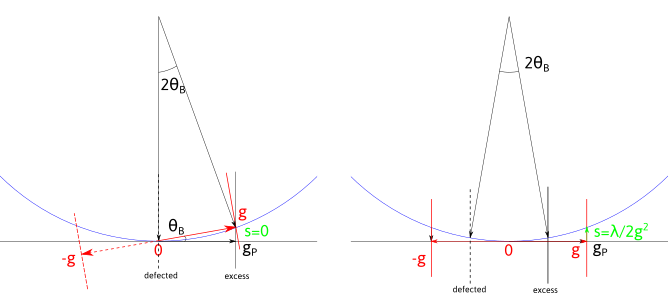A high accuracy of orientation thus needs the knowledge of $s$, i.e. the position of the Kikuchi lines. Please refer to Kikuchi, for such analysis.

When taking a diffraction pattern, it is thus recommended to fulfill the three-beam conditions, i.e. in the middle of the extinction contours in image mode. However better imaging conditions are reached in two-beam with $s>0$.

Pycotem allows to correct the orientation of a diffraction vector from a two-beam condition. See below.

### Calibration and operating conditions¶

The diffraction pattern needs to be carefully calibrated on the microscope used. Use a crystal with known lattice parameters and record a diffraction pattern, ideally forming rows or a zone axis with a large value of $n$. For every camera length and acceleration voltage, measure $r$ in pixels corresponding to $d$ in angstroms.

The calibration are set in the calibration.txt file following the scheme:

Microscope_name voltage camera length binning r.d
...


For Diffraction, both the value $r . d$ and the acceleration voltage (in kV) are necessary, the other fields are informative.

## Orientation from a set of diffraction vectors¶

If a set of diffraction vectors are given, the orientation can be retrieved knowing the position of the vectors in the microscope coordinates $M$ (see below regarding ambiguities). Given the direction $X$ in the crystal $C$ coordinates:

G_C X = G_M \begin{pmatrix} 1\\ 0 \\ 0 \end{pmatrix}

where

G_C=D^\ast \begin{pmatrix} h_1 & k_1 & l_1\\ & ... & \\ h_N & k_N & l_N \end{pmatrix}

are the g-vectors in the crystal coordinates and $D^\ast$ is defined here, and:

G_M=\begin{pmatrix} x_1 & y_1 & z_1\\ & ... & \\ x_N & y_N & z_N \end{pmatrix}

are the g-vectors in the sample coordinates.

The coordinates $x_i,y_i,z_i$ are obtained by appropriate rotation in the sample coordinates. For a double tilt holder, with no angle between the vertical direction and the $\alpha$-tilt axis, the coordinates of the diffraction vector $i$ is 1:

\begin{pmatrix} x_i\\ y_i \\ z_i \end{pmatrix} = R_x(-\beta_i) R_y(-\alpha_i) R_z(-\eta_i) \begin{pmatrix} 0\\ 1 \\ 0 \end{pmatrix}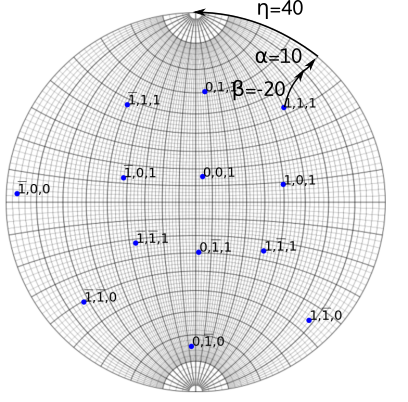Example of a tilt sequence in a double tilt holder. The recorded diffraction vector $(1,1,1)$ is obtained after rotating along $x$ of an angle $\beta=-20$, then along $\alpha=10$. The inclination made on diffraction pattern with the $\alpha$-tilt axis (here along the $y$ direction) is $\eta=40$.

The equations

G_CX=G_M [1,0,0]^T \\ G_CY=G_M [0,1,0]^T \\ G_CZ =G_M [0,0,1]^T

G_C A=G_M

The above equation defines the crystal orientation, so that $A$ should be a rotation matrix. The closest Euler rotation matrix $R$ can be found with the approach proposed by Mackenzie 2 using singular value decomposition:

U,S,V^T=svd(G_C^TG_M) \\ R=V U^T

The Euler angles are retrieved from the $R$ matrix:

\varphi_1 = \arctan (R_{02}, R_{12}) \\ \phi = \arccos(R_{22}) \\ \varphi_2 = \arctan (R_{20},R_{21})

180° ambiguity

When the inputs g-vectors are coplanar, the retrieved orientation may suffer the 180° ambiguity.

The situation described on the left side of the figure below is ambiguous. In this case, the 180° rotation along $g_3$ leads two different crystal orientations. On the contrary, the right side of the figure is not ambiguous, as the 180° rotation along $(0\bar{1}1)$ is a crystal symmetry.

Same ambiguities can arise when indexing zone axes that present a 180° rotational symmetry which does not exist in the crystal.

In Diffraction ambiguous results can be detected.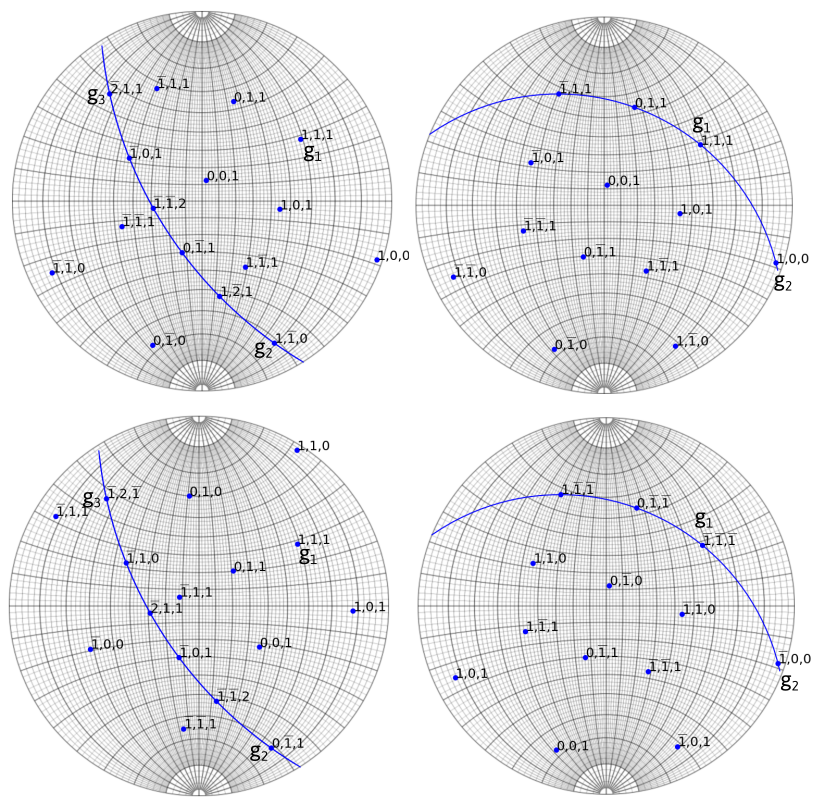## Interface¶

The interface is composed of a top bar menu, a plotting area to display diffraction patterns and a setting/results panel.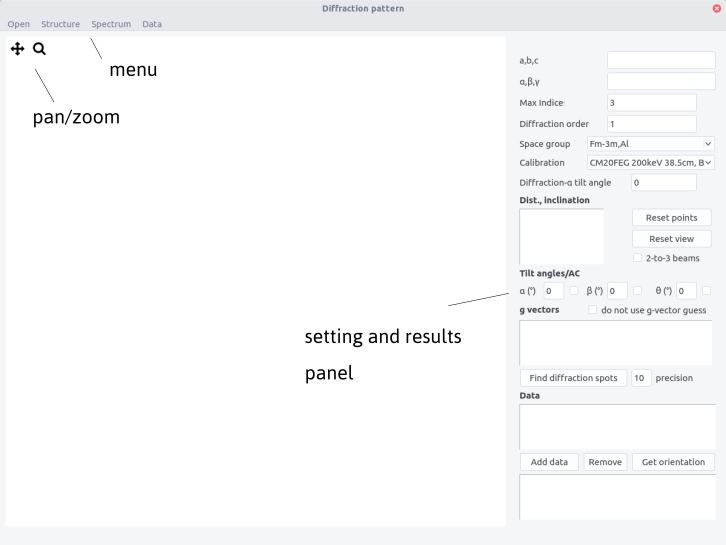## Procedure¶

### Defining the crystal structure¶

Crystal structure can be imported from the structure menu. This menu can be populated by adding structures in the structure.txt files following the template:

Name a(A) b(A) c(A) alpha beta gamma space group


The space group is defined in the space_group.txt file and with the scattering.txt file as defined above.

Alternatively, the structure can be set by filling the $a,b,c$ and $\alpha$, $\beta$, $\gamma$ fields.

### Workflow¶

• Open a diffraction pattern. Make sure that the file name does not contain non-ASCII characters.

• Define a calibration in the Calibrations box

• Set the Diffraction alpha y-axis angle (see stereoproj )

• Left click on a diffraction spot. Zoom in to ensure a higher precision.

• Click again on a second spot corresponding to the diffraction order $n$.

• Indicate the order $n$ in the diffraction order field.

• Click reset view or reset point if needed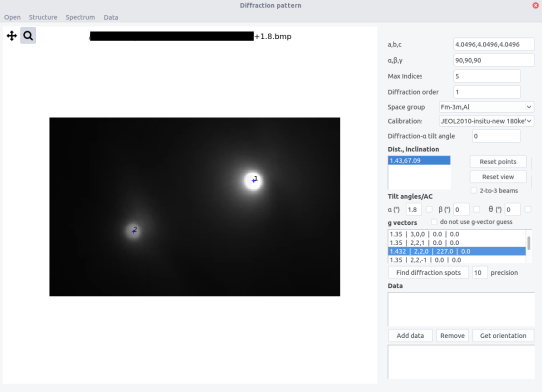• The distance $d$ (A) and the inclination angle $\eta$ are indicated in the Dist, Inclination box.

### Option 1: computer-aided determination of (h,k,l) indices¶

In most of case, the reflection indices can be determined unambiguously from their d-spacing.

• Indicate a value in px in the precision box. The g-vectors will be chosen within the uncertainty range $\pm$ the precision. By default, the value is 10 px.

• Select the d-spacing in the Dist, Inclination box.

• Click on Find diffraction spots to identify the spot. The results appear in the g vectors box with the scheme

d(A) |  h,k,l | Intensity(arb. units) | angles

The angles values are explained below.

The data $(h,k,l)$, the inclination angle $\eta$ and the tilt angles, can be used to plot stereographic projection from a diffraction vector

Thus for every diffraction patterns, the diffraction vector, inclination angle, and tilt angles need to be provided.

• Fill the tilt angles fields. Tick the box to indicate that rotation is anti-clockwise.

• Tick the 2-to-3 beams box if a correction from a 2-beam to a 3-beam condition is required (see above). This leads to the evaluation of the out of plane angle $\epsilon$.

• Click and Find diffraction spots

• Select both the dist, inclination field and the appropriate g vector. For the first condition, the indices of the g-vector can arbitrary be chosen.

• Press Add data. A line appears alpha angle, beta angle, theta angle, inclination, h,k,l, epsilon angle. Data can be removed by selecting the line and by pressing Remove data.

• Repeat the above operations for at least 2 diffraction spots. For every conditions, the angle between the proposed g-vectors and the already chosen g-vectors are indicated. Choose the $(h,k,l)$ indices that minimize all the angles.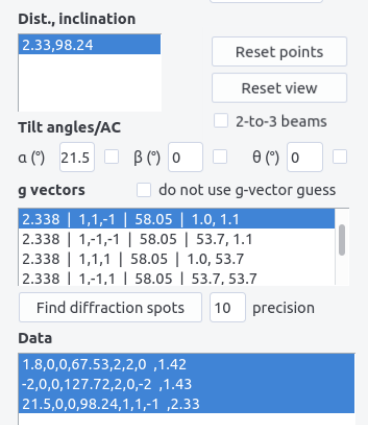### Option 2: Automatic determination¶

When the reflections are ambiguous from their d-spacing, consistent sets of data can be retrieved by comparing the measured angles $\theta_{ij}=\angle{(\vec{g}_i,\vec{g}_j)}$ with a lookup table compiling the angles $\theta'_{ij}=\angle{((h_i,k_i,l_i),(h_j,k_j,l_j))}$. The sets of g-vectors are obtained if all the angle differences $\left|\theta_{ij}-\theta'_{ij}\right|$ is less than a tolerance angle equals to 3 degrees. To proceed this way:

• Tick the do not use g-vector guess box.

• Add the spot as above. The indices will appear as 0,0,0.

### Retrieve orientation¶

• Select the data lines and press Get orientation

The result box shows:

phi1, phi, phi2 (Euler angles)
mean angular deviation, mean d-spacing deviation

In case of option 2, the $(hkl)$ indices are indicated.

Two sets of phi1, phi, phi2 angles are displayed if the results are ambiguous.

The accuracy of the result is given by:

• The mean angular deviation, which is the average angle between each diffraction vector computed from the Euler angles and the experimental values:
\langle \theta_d \rangle = \frac{1}{N}{\sum_i^N \left| \arccos(R \vec{g}_i \cdot [x_i,y_i,z_i]^T) \right|}

where $\vec{g}_i$ is the normalized diffraction vector corresponding to the $(h_i,k_i,l_i)$ plane.

• The mean d-spacing deviation, in percents, is the mean spread between measured and expected d-spacings. It should be of typically less than 10/%
\langle d \rangle= \frac{1}{N}{\sum_i^N \left| \frac{d_{hkl}-d_i}{d_{hkl}}\right|}
• In case of option 2, the results are sorted by ascending order of mean deviation angles and mean d-spacings.## Import/Export data¶

Using the Data menu, data can be imported and results exported. Data sets are provided in a .txt file using the following scheme

# Diffraction data file
alpha_1,beta_1,theta_1,eta_1,h_1,k_1,l_1,epsilon_1
...
alpha_N,beta_N,theta_N,eta_N,h_N,k_N,l_N,epsilon_N


Exporting data will write in a .txt file, adding to the above datasets:

# Results
# Phi1,Phi,Phi2
# phi_1,phi, phi_2
# Mean angular deviation, Mean square angular dev.
# ang_d, ang_d^2

The two files can be combined in one as # sign will not be read during import.

## Spectrum¶

Spectra, i.e. peak intensity with respect to the d-spacing, can be plotted from the top menu. The maximum indices should be indicated.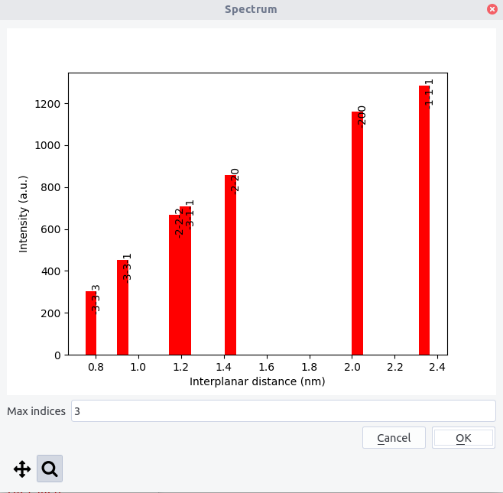1. if the diffraction is taken away from the three-beam condition, an extra rotation $R_x(\epsilon)$ applies, with $\epsilon = \theta_B+s/\|\vec{g}\|^2$

2. J. Mackenzie, Acta Cryst. 1957, 10, 61.